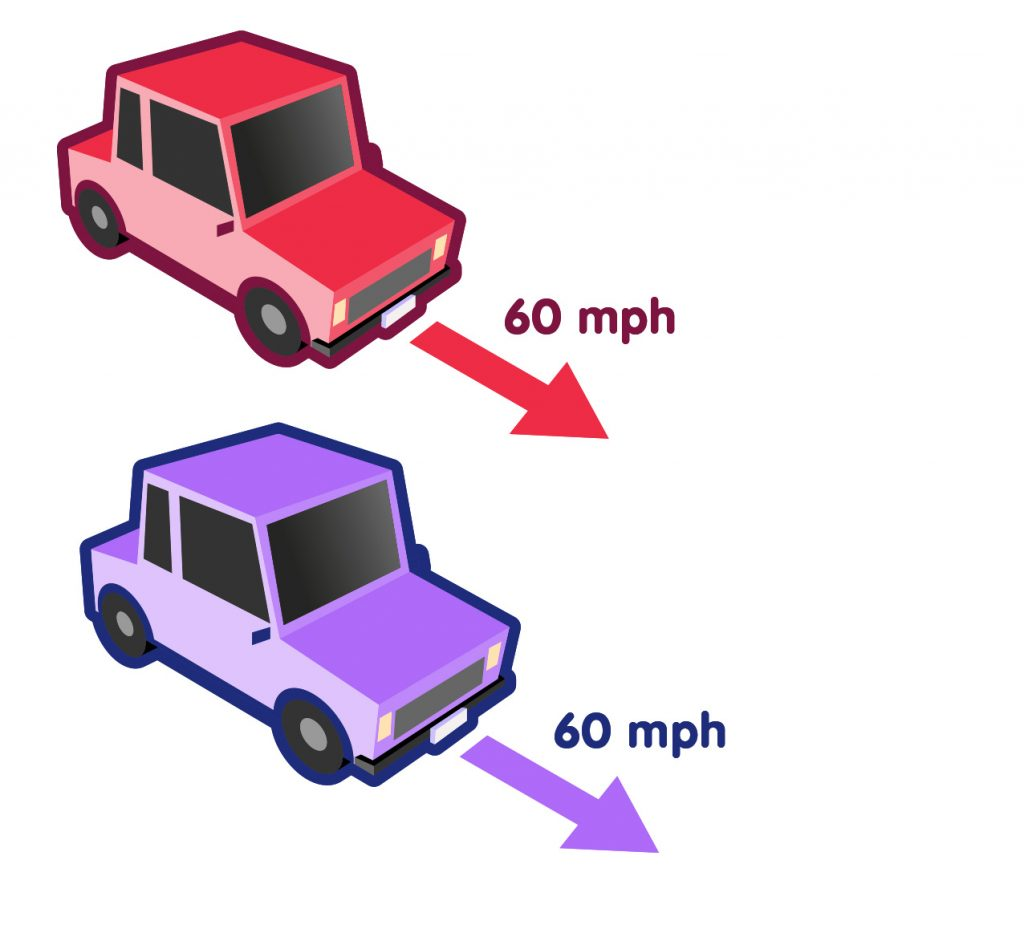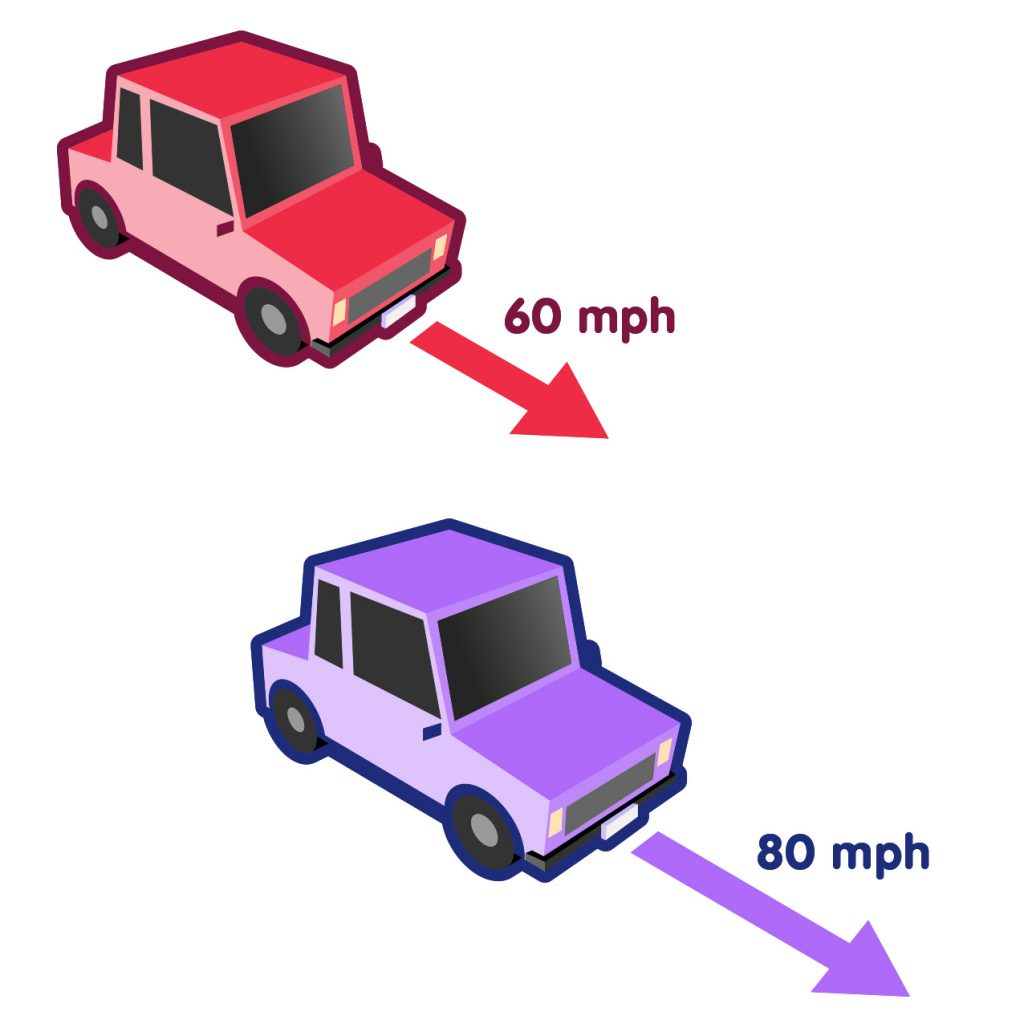# Special relativity / Elementary Tour part 4: The speed of light

On the previous pages, relativity reigned supreme. Although we usually think of lengths and times as absolute, they turned out to be observer-dependent. On this page, the shoe is on the other foot. Ordinarily, we think of velocities as relative, but one of them turns out to be absolute: the speed of light.

From our everyday experience, we’re used to adding or subtracting velocities. Imagine that you’re standing near a highway, watching the cars go by. Let’s say that there are two cars, side by side, each of them with a speed of 60 miles per hour:Two cars driving side by side, each with 60 mph

You would conclude (and rightly so) that from the point of view of each of the drivers, the other car does not move at all. If, on the other hand, one of the cars drove along at 80 miles per hour,Two cars driving side by side, one with 80, the other with 60 mph

then the driver in the other vehicle would see it forge ahead with a speed of 20 miles per hour, relative to his own car. In both cases, speed is subtracted from speed to give relative speed: 60 minus 60 miles per hour gives a relative speed of zero miles per hour in the first case, and 80 minus 60 miles per hour gives 20 miles per hour in the second case.

For light, it would be natural to expect that one could similarly add and subtract velocities. Suppose that I measure a particular light signal’s speed, and find the usual value of 299,792.458 kilometres (186,000 miles) per second. If I see a fast spaceship chase right after that signal, moving at half the speed of light (c/2), I would expect that an observer on that spaceship would measure the speed of my light signal at merely c – c/2 = c/2, half the value that I measured.

Not so, according to special relativity! Simply subtracting speeds would only give the correct answer if the observer on that space-ship measured space and time, distance and duration in the same way that I do. As we have seen on the previous page, that’s not the case. From my point of view, for instance, the measuring rods on the speeding spaceship are shorter than my own, and its clocks run more slowly than mine. Taken together, all of these relativistic effects combine in precisely the right way to result in a surprising phenomenon: Even from the point of view of an observer on the speeding spaceship, my light signal moves with exactly the same speed, c = 299,792.458 kilometres per second.

This phenomenon is far from coincidental. In fact, it is a systematic feature of special relativity. All the different relativistic effects combine, quite generally, so that the following postulate holds true: For any observer on one of the space stations (for any inertial observer, in short), any light signal moves through empty space with the same constant speed, c = 299,792,458 kilometres per second, independent of the motion of the light source.

The speed of light is the only speed that is, in this sense, independent of the observer and thus absolute. It also plays the lead role in all of special relativity.

First of all, it defines the absolute speed limit for the transfer of energy, matter and information. No object, however strong the forces acting upon it, can ever be accelerated to light speed. (Some reasons why that is so are given in the section E = mc².)

Secondly, the speed of light c is a ubiquitous parameter in the equations of special relativity. If the relative speed v is small compared to c, so are the relativistic effects. With v close to c, the relativistic effects become prominent, but with speeds small compared to c, such as the speeds we encounter in everyday life, relativistic effects are almost unnoticable.